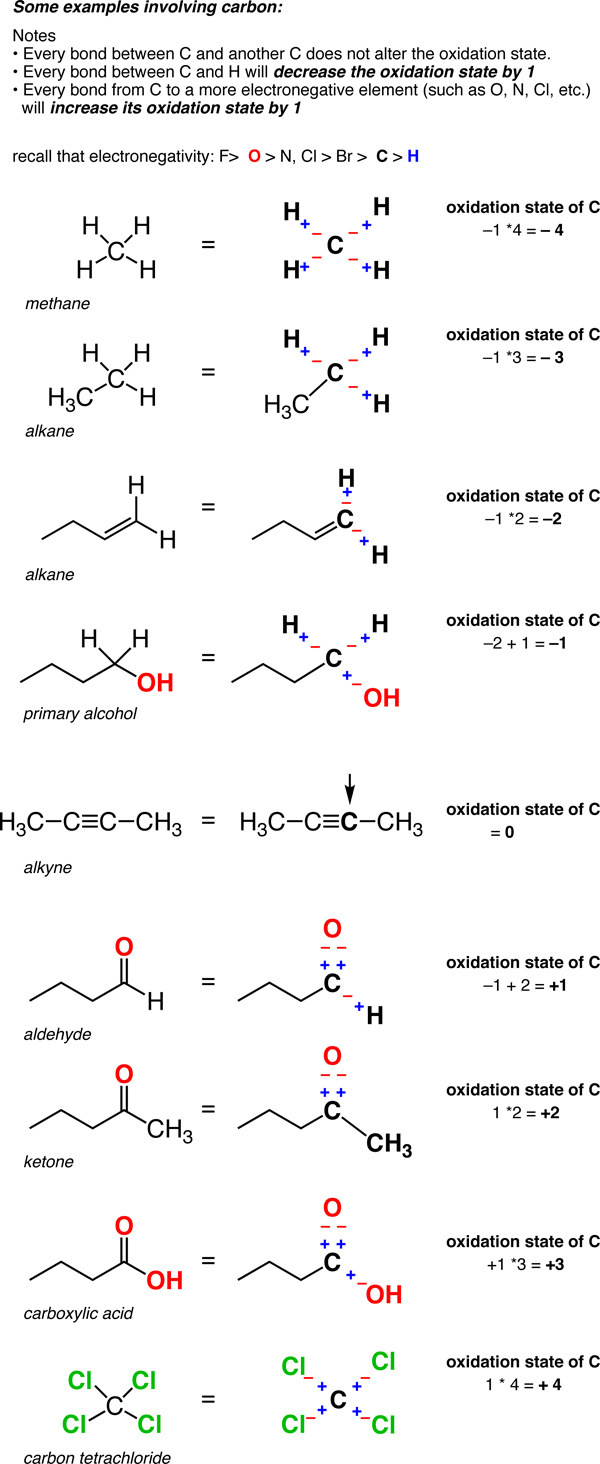# Oxidation States of Organic Molecules

By the end of gen chemistry, calculating oxidation states of different metals should be pretty familiar. Here’s what you do. Take a typical compound – $$FeCl_3$$, for instance. Treat every bond between the metal and a different atom as if it were an ionic bond. That means the more electronegative elements (like chlorine, say, or oxygen) bear negative charges, and the less electronegative element (such as the metal) bears the positive charge.

If the compound is neutral, the sum of the oxidation states also has to be neutral. (If the compound has a charge, you adjust the oxidation states accordingly so that their sum equals the charge).Now here’s a fun exercise. Try applying the same rules to carbon. It’s going to feel a little bit weird. Why? Because there are two key differences:

• First, carbon is often more electronegative (2.5) than some of the atoms it’s bound to (such as H, 2.2). So what do you do in this case?
• Secondly, unlike metal-metal bonds, carbon-carbon bonds are ubiquitous. So how do you deal with them?

1. In a C-H bond, the H is treated as if it has an oxidation state of +1. This means that every C-H bond will decrease the oxidation state of carbon by 1.
2. Any two bonds between the same atom do not affect the oxidation state (recall that the oxidation state of Cl in Cl-Cl (and that of H in H-H) is zero. So a carbon attached to 4 carbons has an oxidation state of zero.

So unlike metals, which are almost always in a positive oxidation state, the oxidation state of carbon can vary widely, from -4 (in CH4) to +4 (such as in CO2). Here are some examples.(Don’t forget that this is called a “formalism” for a reason. The charge on the carbon is not really +4 or –4. But the oxidation state formalism helps us keep track of where the electrons are going, which will come in handy very soon).

With an understanding of how to calculate oxidation states on carbon, we’re ready for the next step: understanding changes in the oxidation state at carbon, through reactions known as oxidations (where the oxidation state is increased), and reductions (where the oxidation state is reduced). More on that next time.